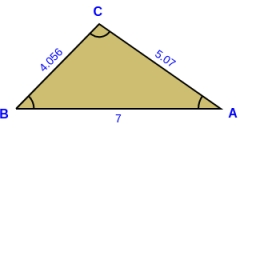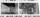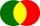# Calculate

Calculate the area of the ABE triangle
AB = 38mm
and height E = 42mm

ps: please try a quick calculation

Correct result:

S =  798 mm2

#### Solution:We would be pleased if you find an error in the word problem, spelling mistakes, or inaccuracies and send it to us. Thank you!Tips to related online calculators

#### You need to know the following knowledge to solve this word math problem:

We encourage you to watch this tutorial video on this math problem:

## Next similar math problems:

• Edge cFind the edge c of cuboid if an edge a = 20 mm, b = 30 mm and surface area S = 8000 mm2.
• ShapePlane shape has a maximum area 677 mm2. Calculate its perimeter if perimeter is the smallest possible.
• Right triangleRight triangle legs has lengths 630 mm and 411 dm. Calculate the area of this triangle.
• RainfallHow many liters of water did fell in a 32m long and 8m wide garden, if 8mm of rain fell?
• Triangle ABCConstruct a triangle ABC is is given c = 60mm hc = 40 mm and b = 48 mm analysis procedure steps construction
• The rawThe raw data presented here are the scores (out of 100 marks) of a market survey regarding the acceptability of new product launched by a company for random sample of 50 respondents: 40 45 41 45 45 30 30 8 48 25 26 9 23 24 26 29 8 40 41 42 39 35 18 25 35
• Isosceles trapezoidFind the area of an isosceles trapezoid with bases of 8cm and 72mm. The height of the trapezoid is equal to three-quarters of the longer base.
• Photo egativeNegative dimensions are 36mm and 28mm. What will be the photo size in the 21:4 ratio?
• Triangular prismCalculate a triangular prism if it has a rectangular triangle base with a = 4cm and hypotenuse c = 50mm and height of the prism is 0.12 dm.
• Area 4gonCalculate area of 4-gon, two and the two sides are equal and parallel with lengths 11, 5, 11 and 5. Inner angles are 45°, 135°,45°, 135°.
• Rhombus 2Calculate the rhombus area, which has a height v=48 mm and shorter diagonal u = 60 mm long.
• IntersectionsHow many intersections have circles with radius 16 mm and 15 mm, if the distance of their centers is 16 mm.
• Height to the baseThe triangle area is 35 cm ^ 2. The size of the base is 10 cm. Find the length of height to the base.
• Plan of the villagePlan of the municipality in 1:1000 scale has plotted garden with dimensions 25 mm and 28 mm. Determine the area of gardens in ares in reality.
• Pyramid four sidesIn a regular tetrahedral pyramid is a body height 38 cm and a wall height 42 cm. Calculate the surface area of the pyramid; the result round to square centimeters.
• Reverse Pythagorean theoremGiven are lengths of the sides of the triangles. Decide which one is rectangular: Δ ABC: 77 dm, 85 dm, 36 dm ? Δ DEF: 55 dm, 82 dm, 61 dm ? Δ GHI: 24 mm, 25 mm, 7 mm ? Δ JKL: 32 dm, 51 dm, 82 dm ? Δ MNO: 51 dm, 45 dm, 24 dm ?
• 2d shapeCalculate the content of a shape in which an arbitrary point is not more than 3 cm from the segment AB. The length of the segment AB is 5 cm.